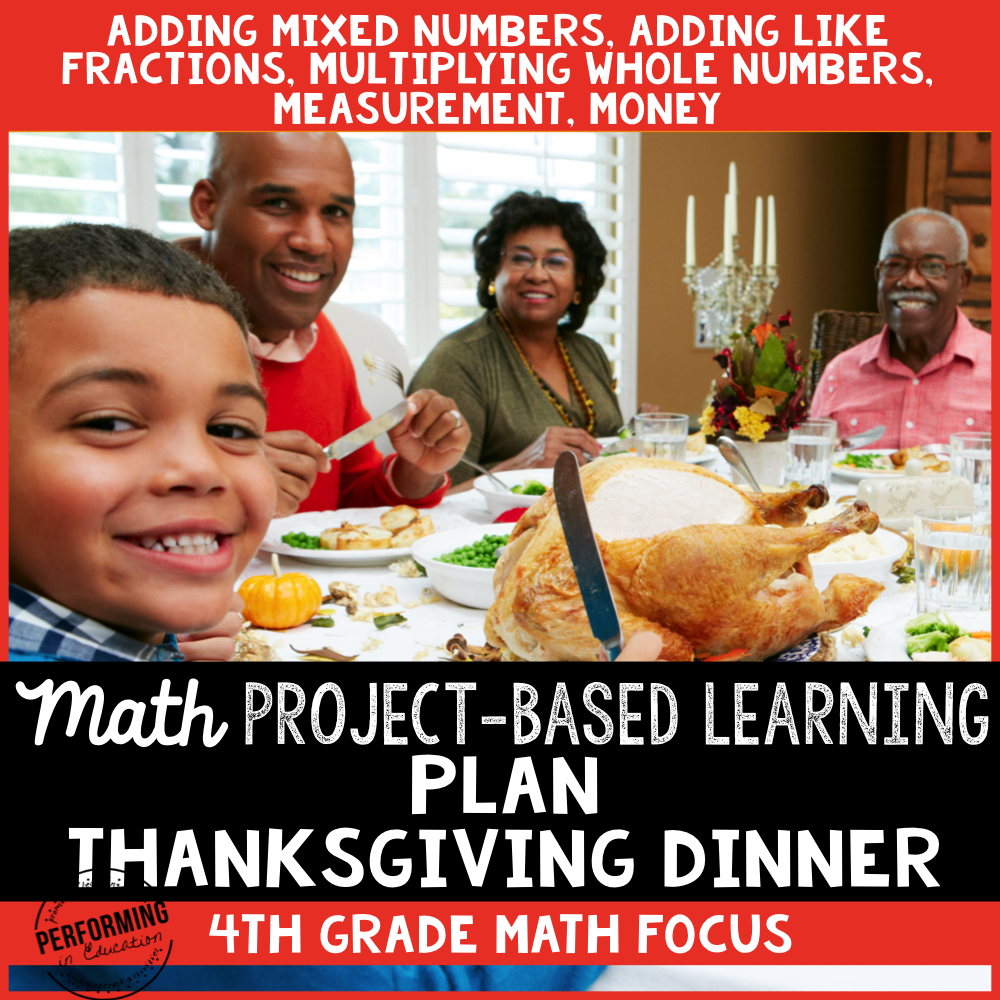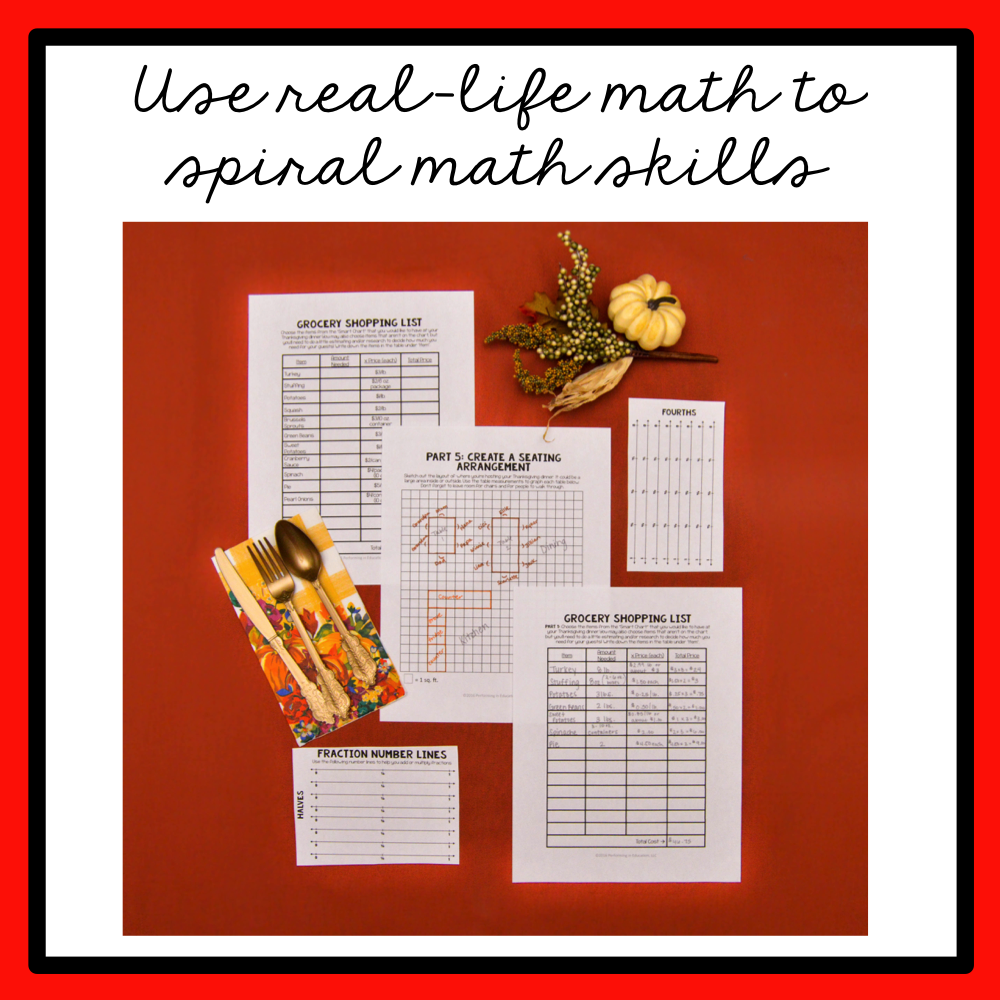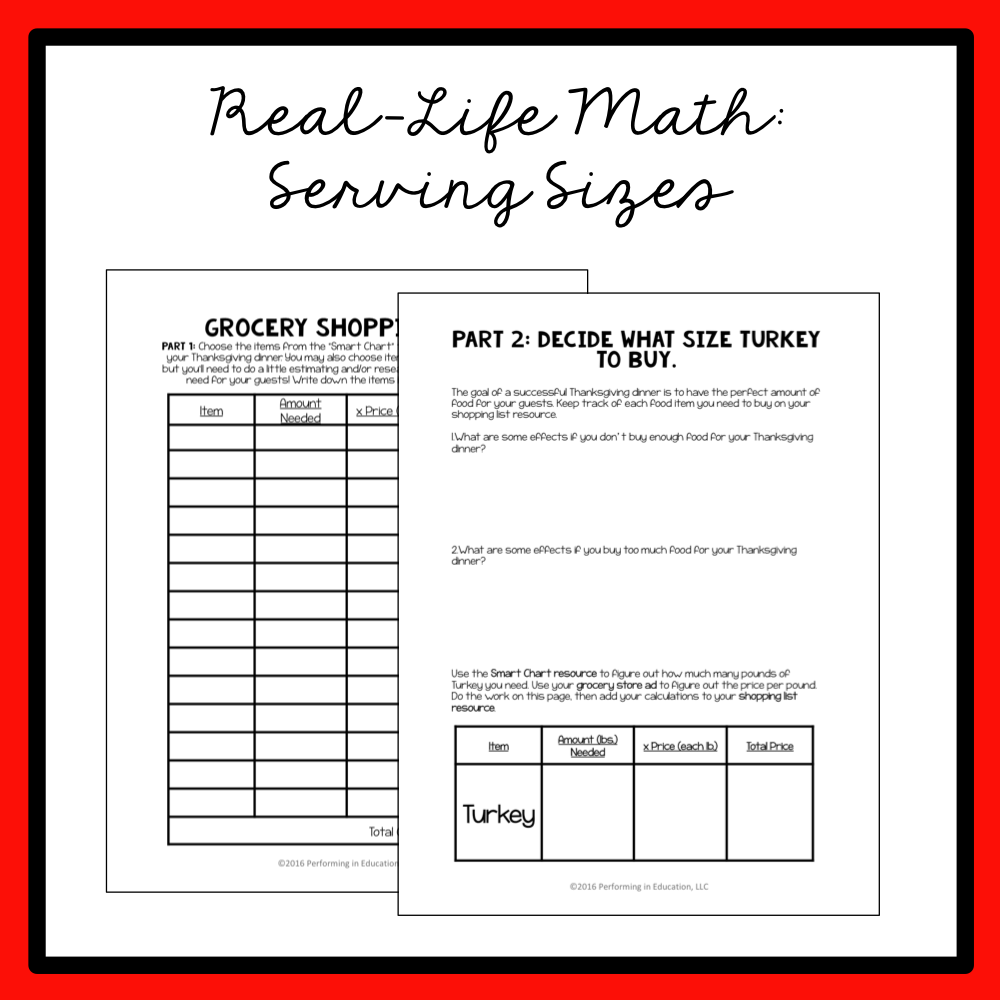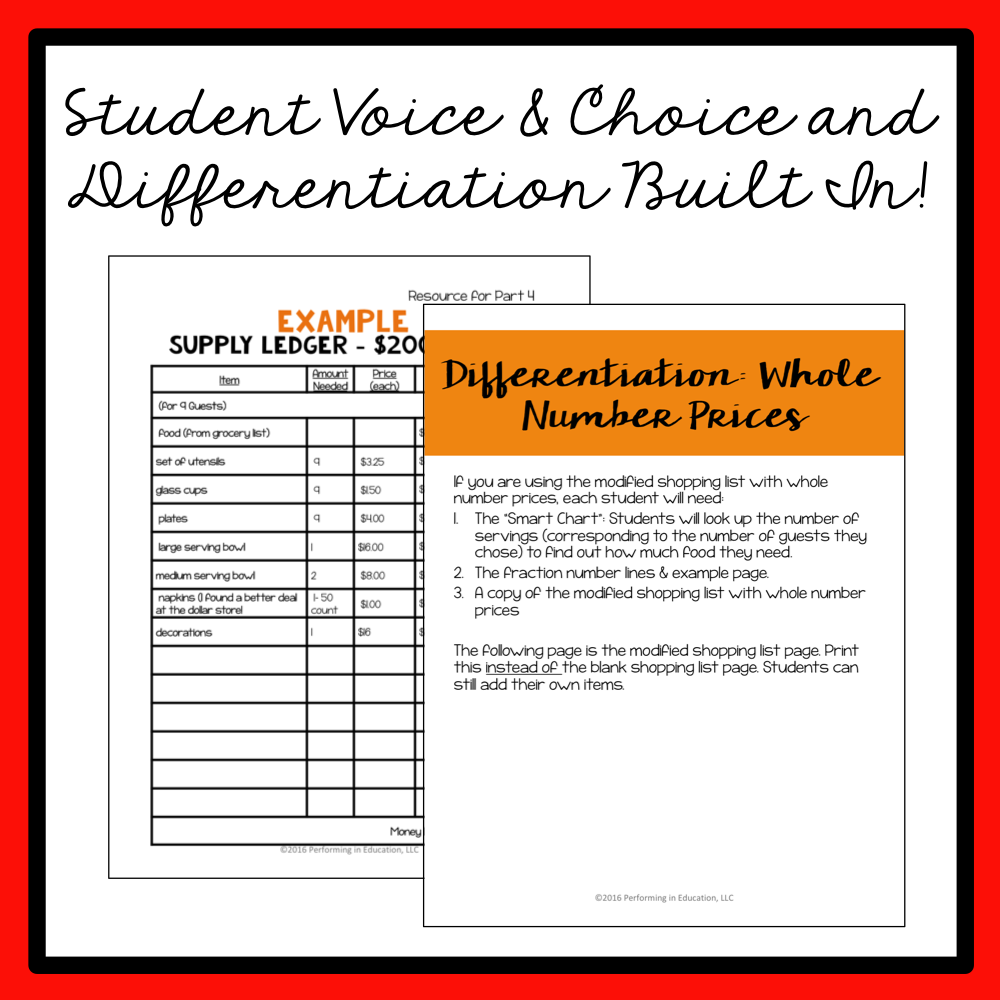# Thanksgiving Project-Based Learning for 4th Grade Fractions & Measurement

00048Have your 4th graders plan Thanksgiving Dinner in this fun Math Project-based learning activity! Standards covered include adding mixed numbers, adding like fractions, multiplying fractions by whole numbers, multiplying whole numbers, measurement (conversion from inches to feet), and money. Great for Common Core Math. Help your students practice real-life and math skills with this quality math project. It includes a teacher guide walking you through each step. Differentiation and student choice is built into all of my PBLs!

Your students will be SO excited to do math during this ENGAGING project. Students will use ALL mathematical practices and learn real-world math skills.

Apply The following math skills:

• adding mixed numbers
• adding like fractions
• multiplying fractions by whole numbers
• multiplying whole numbers
• measurement (conversion from inches to feet)
• money

All of my project-based learning activities come with free e-mail support!

\$5 In stock